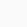# How to use not equal(≠) symbol in LaTeX?

Symbol/UnicodeNot equal/U+2260
Type of symbolInequality
Package (requirement)No
ArgumentNo
Latex command\ne
Example\ne → ≠

The most popular symbol used in mathematics is “not equal”. In Latex, you need to use \ne command for this symbol, you can also use \neq command.

\documentclass{article}
\begin{document}
$a \ne b$
$\cos x \ne \sin x$
% Use \neq command
$5 \neq 3$
$x^2 + 1 \neq y^2 + 1$
\end{document}

Output :Scroll to Top